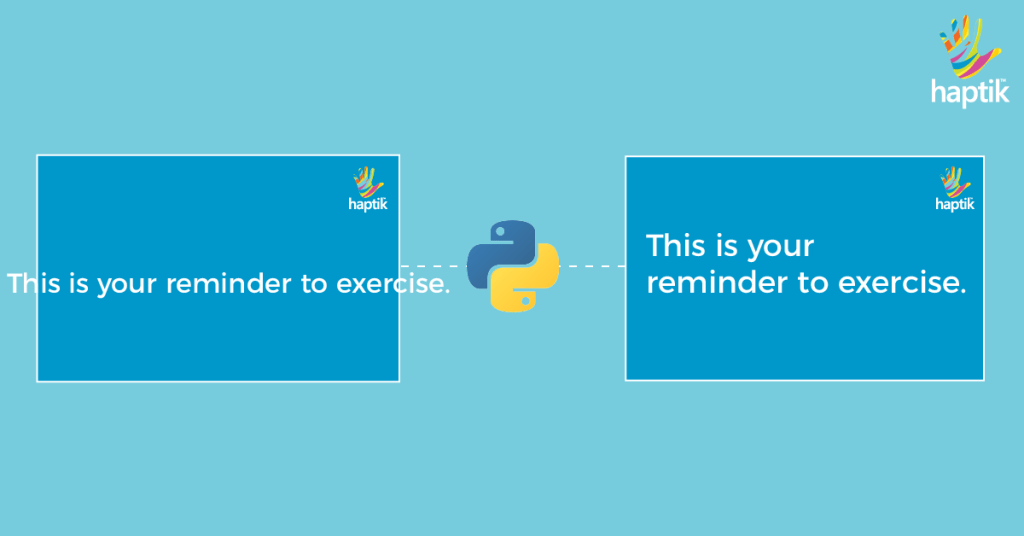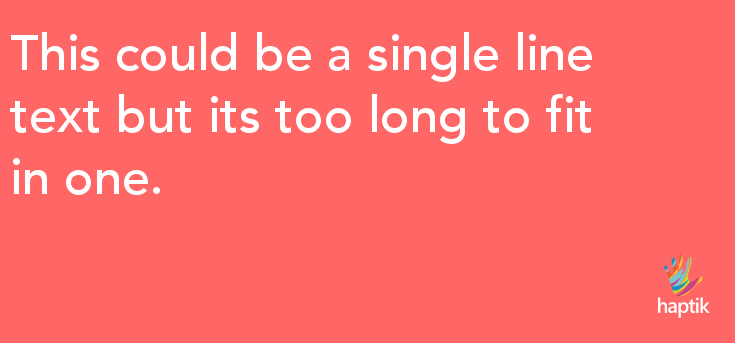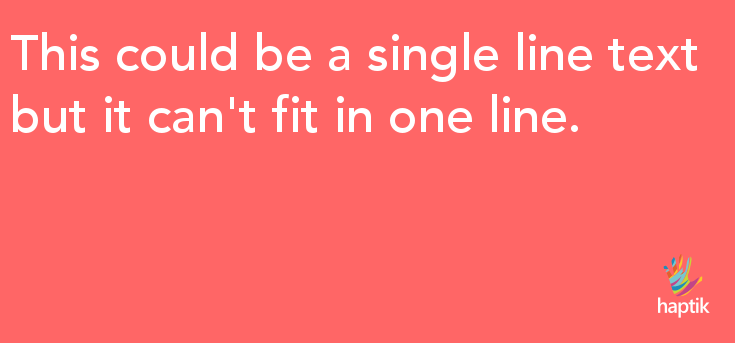In the first post of this series, I introduced you to the basics of text drawing in Python by adding a greeting text on an image. I also highlighted examples of how I further extended this functionality to create some complex images at work.

If you haven’t already read the first part of this series (Putting Text on Images Using Python Part -1), I recommend you take a glance at it first, to get a better understanding of this post.

For now, we know how to draw text, change the font, and position the text on the image. In this post, we’ll discover how to draw multiline text and also discuss the challenges of doing so.### Multiline Text

Often, while generating images, we come across situations where the text doesn’t fit in a single line. Python Pillow is not helpful here as it doesn’t automatically draw & push the text to a new line. In order to do this manually, we need to calculate the width and height of the text.

With the text-width, we determine when we need to move to the next line and with the text-height, we can figure how much space should be left in between the two lines:The idea is to split the long sentences into multiple shorter sentences and draw each of these, one by one at the correct positions thereby making it look like a multiline text. To split a longer line, we‘ll use a Pillow function to calculate the size of the text passed to it as one of the parameters.

### Calculate Text Width

For convenience, I’ve created a method text_wrap() to explain the line-split logic:

 `from PIL import Image` `from PIL import ImageFont` `from PIL import ImageDraw` ` ` `def text_wrap(text, font, max_width):` `    lines = []` `    # If the width of the text is smaller than image width` `    # we don't need to split it, just add it to the lines array` `    # and return` `    if font.getsize(text) <= max_width:` `        lines.append(text) ` `    else:` `        # split the line by spaces to get words` `        words = text.split(' ')  ` `        i = 0` `        # append every word to a line while its width is shorter than image width` `        while i < len(words):` `            line = ''         ` `            while i < len(words) and font.getsize(line + words[i]) <= max_width:                ` `                line = line + words[i] + " "` `                i += 1` `            if not line:` `                line = words[i]` `                i += 1` `            # when the line gets longer than the max width do not append the word, ` `            # add the line to the lines array` `            lines.append(line)    ` `    return lines` ` ` ` ` `def draw_text(text):    ` `    # open the background file` `    img = Image.open('background.png')` `    ` `    # size() returns a tuple of (width, height) ` `    image_size = img.size ` ` ` `    # create the ImageFont instance` `    font_file_path = 'fonts/Avenir-Medium.ttf'` `    font = ImageFont.truetype(font_file_path, size=50, encoding="unic")` ` ` `    # get shorter lines` `    lines = text_wrap(text, font, image_size)` `    print lines # ['This could be a single line text ', 'but its too long to fit in one. ']` ` ` ` ` `if __name__ == __main__:` `    draw_text("This could be a single line text but its too long to fit in one.")`

This function expects three parameters – the text to draw, an ImageFont class instance and the width of the background image on which the text is to be drawn.

The logic is pretty straightforward:

1. Check, if the sentence can fit in one line then just return it without splitting, else:
2. Split the sentence using spaces to fetch the words in it
3. Create shorter lines by appending words while the width is smaller than the image width

When we run this script it returns an array containing 2 shorter lines which fit within the width of the background image.

To draw these lines on the image we have to calculate the correct vertical position of each line.

### Calculate Text Height

Whenever we write text, there is an equal amount of space between two lines. For example in this post, the lines have the constant spaces between them. While building this library I faced an issue of varying spaces with most of the input text:

 `text = "This could be a single line text but it can't fit in one line."` `lines = text_wrap(lines, font)` ` ` `for line in lines:` `    print font.getsize(line)` ` ` `# Output` `# 62` `# 51`

Finding correct height for characters like g, j, p, q, y which are drawn below the Baseline and b, d, f, h, k, l  which are drawn above the Median is a little tedious due to varying heights.The best way to get the correct height of the text is to simply calculate the total height of “hg”. This trick works because h and g cover the height range of all the English characters.

For languages other than English, you might have to use different characters in place of & g.

 `text = "This could be a single line text but it can't fit in one line."` `lines = text_wrap(lines, font)` ` ` `line_height = font.get_size('hg')` `print line_height` ` ` `# Output` `# 62`

Since we have our wrapped/short lines and also the text height we can draw these on the image. We can do this by keeping a reference to the vertical position of the previously drawn line and then adding to it the line height to calculate the vertical position of the new line:

 `text = "This could be a single line text but its too long to fit in one."` `lines = text_wrap(text, font, image_size)` `line_height = font.getsize('hg')` ` ` `x = 10` `y = 20` `for line in lines:` `    # draw the line on the image` `    draw.text((x, y), line, fill=color, font=font)` `    ` `    # update the y position so that we can use it for next line` `    y = y + line_height` `# save the image` `img.save('word2.png', optimize=True)`

This will output an image like this:The text in the latter images looks much better and readable. At Haptik, we believe in experimentation and finding out the best possible way to solve problems. The above is one such example. In my next Blog post, I will be writing about how to center align text horizontally and vertically in an image using Python.

Think we did a good job? Let us know in the comments below.

Also, Haptik is hiring. Visit our careers section or get in touch with us at hello@haptik.ai.

View All